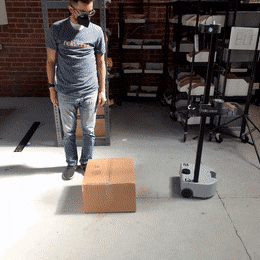# Mobile Base Collision Avoidance

## Example 3

This example aims to combine the two previous examples and have Stretch utilize its laser scan data to avoid collision with objects as it drives forward.

Begin by running the following command in a new terminal.

``````roslaunch stretch_core stretch_driver.launch
``````

Then, in a new terminal, type the following to activate the LiDAR sensor.

``````roslaunch stretch_core rplidar.launch
``````

To set `navigation` mode and to activate the avoider.py node, type the following in a new terminal.

``````rosservice call /switch_to_navigation_mode
cd catkin_ws/src/stretch_tutorials/src/
python3 avoider.py
``````

To stop the node from sending twist messages, type `Ctrl` + `c` in the terminal running the avoider node.### The Code

``````#!/usr/bin/env python3

import rospy
from numpy import linspace, inf, tanh
from math import sin
from geometry_msgs.msg import Twist
from sensor_msgs.msg import LaserScan

class Avoider:
"""
A class that implements both a LaserScan filter and base velocity control
for collision avoidance.
"""
def __init__(self):
"""
Function that initializes the subscriber, publisher, and marker features.
:param self: The self reference.
"""
self.pub = rospy.Publisher('/stretch/cmd_vel', Twist, queue_size=1) #/stretch_diff_drive_controller/cmd_vel for gazebo
self.sub = rospy.Subscriber('/scan', LaserScan, self.set_speed)

self.width = 1
self.extent = self.width / 2.0
self.distance = 0.5

self.twist = Twist()
self.twist.linear.x = 0.0
self.twist.linear.y = 0.0
self.twist.linear.z = 0.0
self.twist.angular.x = 0.0
self.twist.angular.y = 0.0
self.twist.angular.z = 0.0

def set_speed(self,msg):
"""
Callback function to deal with incoming LaserScan messages.
:param self: The self reference.
:param msg: The subscribed LaserScan message.

:publishes self.twist: Twist message.
"""
angles = linspace(msg.angle_min, msg.angle_max, len(msg.ranges))
points = [r * sin(theta) if (theta < -2.5 or theta > 2.5) else inf for r,theta in zip(msg.ranges, angles)]
new_ranges = [r if abs(y) < self.extent else inf for r,y in zip(msg.ranges, points)]
error = min(new_ranges) - self.distance

self.twist.linear.x = tanh(error) if (error > 0.05 or error < -0.05) else 0
self.pub.publish(self.twist)

if __name__ == '__main__':
rospy.init_node('avoider')
Avoider()
rospy.spin()
``````

### The Code Explained

Now let's break the code down.

``````#!/usr/bin/env python3
``````

Every Python ROS Node will have this declaration at the top. The first line makes sure your script is executed as a Python3 script.

``````import rospy
from numpy import linspace, inf, tanh
from math import sin
from geometry_msgs.msg import Twist
from sensor_msgs.msg import LaserScan
``````

You need to import `rospy` if you are writing a ROS Node. There are functions from `numpy` and `math` that are required within this code, thus `linspace`, `inf`, `tanh`, and `sin` are imported. The `sensor_msgs.msg` import is so that we can subscribe to `LaserScan` messages. The `geometry_msgs.msg` import is so that we can send velocity commands to the robot.

``````self.pub = rospy.Publisher('/stretch/cmd_vel', Twist, queue_size=1)#/stretch_diff_drive_controller/cmd_vel for gazebo
``````

This section of the code defines the talker's interface to the rest of ROS. `pub = rospy.Publisher("/stretch/cmd_vel", Twist, queue_size=1)` declares that your node is publishing to the `/stretch/cmd_vel` topic using the message type `Twist`.

``````self.sub = rospy.Subscriber('/scan', LaserScan, self.set_speed)
``````

Set up a subscriber. We're going to subscribe to the topic `scan`, looking for `LaserScan` messages. When a message comes in, ROS is going to pass it to the function `set_speed()` automatically.

``````self.width = 1
self.extent = self.width / 2.0
self.distance = 0.5
``````

`self.width` is the width of the laser scan we want in front of Stretch. Since Stretch's front is pointing to the x-axis, any points with y coordinates further than half of the defined width (self.extent) from the x-axis are not considered. `self.distance` defines the stopping distance from an object.

``````self.twist = Twist()
self.twist.linear.x = 0.0
self.twist.linear.y = 0.0
self.twist.linear.z = 0.0
self.twist.angular.x = 0.0
self.twist.angular.y = 0.0
self.twist.angular.z = 0.0
``````

Allocate a `Twist` to use, and set everything to zero. We're going to do this when the class is initiated. Redefining this within the callback function, `set_speed()` can be more computationally taxing.

``````angles = linspace(msg.angle_min, msg.angle_max, len(msg.ranges))
points = [r * sin(theta) if (theta < -2.5 or theta > 2.5) else inf for r,theta in zip(msg.ranges, angles)]
new_ranges = [r if abs(y) < self.extent else inf for r,y in zip(msg.ranges, points)]
``````

This line of code utilizes `linspace` to compute each angle of the subscribed scan. Here we compute the y coordinates of the ranges that are below -2.5 and above 2.5 radians of the scan angles. These limits are sufficient for considering scan ranges in front of Stretch, but these values can be altered to your preference. If the absolute value of a point's y-coordinate is under `self.extent` then keep the range, otherwise use `inf`, which means "no return".

``````error = min(new_ranges) - self.distance
``````

Calculate the difference of the closest measured scan and where we want the robot to stop. We define this as `error`.

``````self.twist.linear.x = tanh(error) if (error > 0.05 or error < -0.05) else 0
``````

Set the speed according to a `tanh` function. This method gives a nice smooth mapping from distance to speed, and asymptotes at +/- 1

``````self.pub.publish(self.twist)
``````

Publish the `Twist` message.

``````rospy.init_node('avoider')
Avoider()
rospy.spin()
``````

The next line, `rospy.init_node(NAME, ...)`, is very important as it tells rospy the name of your node -- until rospy has this information, it cannot start communicating with the ROS Master.

Note

The name must be a base name, i.e. it cannot contain any slashes "/".

Instantiate class with `Avioder()`.

Give control to ROS with `rospy.spin()`. This will allow the callback to be called whenever new messages come in. If we don't put this line in, then the node will not work, and ROS will not process any messages.